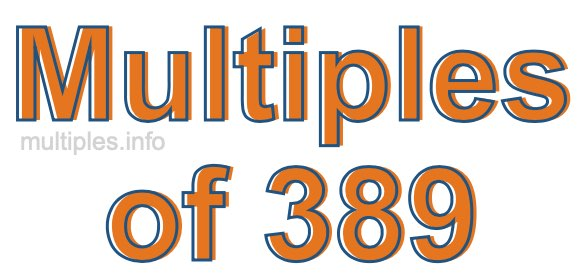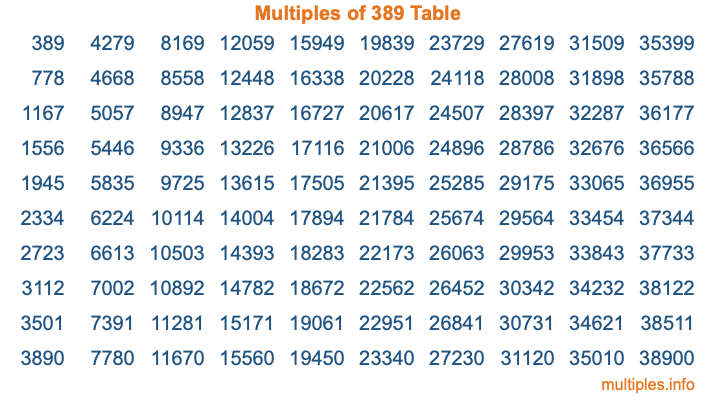Multiples of 389Welcome to the Multiples of 389 page. Here we will first teach you everything you will ever need to know about the multiples of 389, and then give you a study guide summary of everything we taught you to make sure you remember it all. Use this page to look up facts and learn information about the multiples of 389. This page will make you a multiples of three hundred eighty-nine expert!

Definition of Multiples of 389
Multiples of 389 are all the numbers that when divided by 389 equal an integer. Each of the multiples of 389 are called a multiple. A multiple of 389 is created by multiplying 389 by an integer.

Therefore, to create a list of multiples of 389, you start with 1 multiplied by 389, then 2 multiplied by 389, then 3 multiplied by 389, and so on for as long as you want. Thus, the list of the first five multiples of 389 is 389, 778, 1167, 1556, and 1945. To see a larger list of multiples of 389, see the printable image of Multiples of 389 further down on this page. We also have a category where you can choose any nth multiple of 389.

Multiples of 389 Checker
The Multiples of 389 Checker below checks to see if any number of your choice is a multiple of 389. In other words, it checks to see if there is any number (integer) that when multiplied by 389 will equal your number. To do that, we divide your number by 389. If the the quotient is an integer, then your number is a multiple of 389.

Is  a multiple of 389?

Least Common Multiple of 389 and ...
A Least Common Multiple (LCM) is the lowest multiple that two or more numbers have in common. This is also called the smallest common multiple or lowest common multiple and is useful to know when you are adding our subtracting fractions. Enter one or more numbers below (389 is already entered) to find the LCM.

Check out our LCM Calculator if you need more details about the Least Common Multiple or if you need the LCM for different numbers for adding and subtraction fractions.

nth Multiple of 389
As we stated above, 389 is the first multiple of 389, 778 is the second multiple of 389, 1167 is the third multiple of 389, and so on. Enter a number below to find the nth multiple of 389.

th multiple of 389

Multiples of 389 vs Factors of 389
389 is a multiple of 389 and a factor of 389, but that is where the similarities end. All postive multiples of 389 are 389 or greater than 389. All positive factors of 389 are 389 or less than 389.

Below is the beginning list of multiples of 389 and the factors of 389 so you can compare:

Multiples of 389: 389, 778, 1167, 1556, 1945, etc.

Factors of 389: 1, 389

As you can see, the multiples of 389 are all the numbers that you can divide by 389 to get a whole number. The factors of 389, on the other hand, are all the whole numbers that you can multiply by another whole number to get 389.

It's also interesting to note that if a number (x) is a factor of 389, then 389 will also be a multiple of that number (x).

Multiples of 389 vs Divisors of 389
The divisors of 389 are all the integers that 389 can be divided by evenly. Below is a list of the divisors of 389.

Divisors of 389: 1, 389

The interesting thing to note here is that if you take any multiple of 389 and divide it by a divisor of 389, you will see that the quotient is an integer.

Multiples of 389 Table
Below is an image of the first 100 multiples of 389 in a table. The table is in chronological order, column by column. The first column has the first ten multiples of 389, the second column has the next ten multiples of 389, and so on.The Multiples of 389 Table is also referred to as the 389 Times Table or Times Table of 389. You are welcome to print out our table for your studies.

Negative Multiples of 389
Although not often discussed or needed in math, it is worth mentioning that you can make a list of negative multiples of 389 by multiplying 389 by -1, then by -2, then by -3, and so on, to get the following list of negative multiples of 389:

-389, -778, -1167, -1556, -1945, etc.

Multiples of 389 Summary
Below is a summary of important Multiples of 389 facts that we have discussed on this page. To retain the knowledge on this page, we recommend that you read through the summary and explain to yourself or a study partner why they hold true.

There are an infinite number of multiples of 389.

A multiple of 389 divided by 389 will equal a whole number.

389 divided by a factor of 389 equals a divisor of 389.

The nth multiple of 389 is n times 389.

The largest factor of 389 is equal to the first positive multiple of 389.

389 is a multiple of every factor of 389.

389 is a multiple of 389.

A multiple of 389 divided by a divisor of 389 equals an integer.

389 divided by a divisor of 389 equals a factor of 389.

Any integer times 389 will equal a multiple of 389.

Multiples of a Number
Here you can get the multiples of another number, all with the same attention to detail as we did for multiples of 389 on this page.

Multiples of
Multiples of 390
Did you find our page about multiples of three hundred eighty-nine educational? Do you want more knowledge? Check out the multiples of the next number on our list!